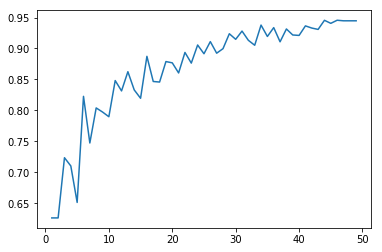Adaboost linearly combines weak learners into a single strong learner in an iterative fashion.
At each iteration, the higher weights are set on previously misclassified samples, a weak learner is trained on such weighted samples

from __future__ import division
import numpy as np
from sklearn.ensemble import AdaBoostClassifier
from sklearn.tree import DecisionTreeClassifier
from sklearn.datasets import make_gaussian_quantiles
from matplotlib.pyplot import plot
%matplotlib inline

X, y = make_gaussian_quantiles(n_samples=10000, n_features=5,n_classes=2, random_state=1)
y[y==0] = -1

def fit(self, X, y, n_estimators):
self.weights = np.array([1/X.shape] * X.shape)
self.learners = []
self.alphas = []

for i in range(n_estimators):
clf = DecisionTreeClassifier(max_depth=2)
clf.fit(X,y,self.weights)
self.learners.append(clf)

e = np.sum(self.weights[np.nonzero(clf.predict(X) != y)])
a = .5 * np.log((1-e)/e)
self.alphas.append(a)

self.weights = self.weights*np.exp(-y*a*clf.predict(X))
self.weights = self.weights/np.sum(self.weights)

return self

def score(self, X, y):
ps = np.zeros(X.shape)

for i in range(len(self.learners)):
l = self.learners[i]
a = self.alphas[i]

ps += a * l.predict(X)

ps[ps < 0] = -1
ps[ps >= 0] = 1

return np.count_nonzero(ps == y) / y.shape

plot(range(1,50), [myAdaBoost().fit(X,y,i).score(X,y)for i in range(1,50)])2017年上半年软考报名时间汇总（持续更新）

liuyiok
2017/02/13
0
0
Day186 | 遇见GCS（七）

7月份说超级节点计划马上开启，不过一个多月过去，还没有消息。 新闻：http://blockchain.game/news.html 开发者社区：http://developer.blockchain.game/ 新版官网上线：http://blockchain...

2018/08/21
0
0
2017年2月教育信息化工作月报

《教育部2017年工作要点》和《教育部科技司2017年工作要点》明确了教育信息化工作任务，教育部制定了《“十三五”国家信息化规划》相关重点任务部内分工方案，组织赴海南、重庆等地开展了中小...

2018/04/16
0
0

DataHunter小数
2018/06/01
0
0
Coffey/JQuery EasyUI 1.5.x Of Insdep Theme

#jQuery EasyUI 1.5.x of insdep theme 下方附部分美化截图 Insdep theme是基于EasyUI 1.5.x 的一款免费的美化主题包，拥有百度编辑器、cropper、Highcharts、justgage、plupload等各类适应本...

Coffey
2017/02/13
0
0

3_数组

3_数组

7
0

APEMESH

7
0

zxiaofan666

10
0
X-MSG-IM-分布式信令跟踪能力

dev5

7
0
OpenJDK之CyclicBarrier

OpenJDK8，本人看的是openJDK。以前就看过，只是经常忘记，所以记录下 图1 CyclicBarrier是Doug Lea在JDK1.5中引入的，作用就不详细描述了，主要有如下俩个方法使用: await()方法，如果当前线...

8
0